## Tamilnadu Samacheer Kalvi 6th Maths Solutions Term 1 Chapter 1 Numbers Ex 1.4Question 1.
Fill in the blanks.
(i) The nearest 100 of 843 is _____
(ii) The nearest 1000 of 756 is ______
(iii) The nearest 10,000 of 85654 is ______
Solution:
(i) 800.
The digit in tens place is 4 < 5
(ii) 1000.
The digit in hundred places is 7 ≥ 5
(iii) 90,000.
The digit in a thousand places is 5 ≥ 5

Question 2.
Say True or False.
(i) 8567 is rounded off as 8600 to the nearest 10.
(ii) 139 is rounded off as 100 to the nearest 100.
(iii) 1,70,51,972 is rounded off as 1,70,00,000 to the nearest lakh.
Solution:
(i) False
(ii) True
(iii) FalseQuestion 3.
Round off the following to the given nearest place.
(i) 4065; hundred
(ii) 44,555; thousand
(iii) 86943; ten thousand
(iv) 50,81,739; lakh
(v) 33,75,98,482; Ten crore
Solution:
(i) We have to round off 4065 to hundreds
The place value is a hundred
The digit in hundreds place is 0
The digit to the right is 6 > 5
Adding 1 to 0 ⇒ 0 + 1 = 1
Changing the digits to the right of 41 to zeros
4065 $$\simeq$$ 4100 ⇒ 4100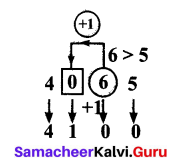(ii) To round off 44555 to thousands
The digit in the thousands place is 4
digit to the right is 5 = 5.
Adding 1 to the thousand place value digit 4 ⇒ 4 + 1 = 5
Changing the digits to the right of 45 to zeros we get 45000
44555 $$\simeq$$ 45000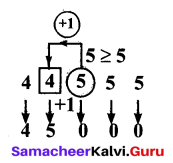(iii) To round off 86,943 to ten thousand
The digit in ten thousand places is 8
The digit to the right is 6 > 5
Adding 1 to 8 ⇒ 8 + 1 = 9.
Changing the digits to the right of 9 to zeroes we get 90,000
86943 $$\simeq$$ 90,000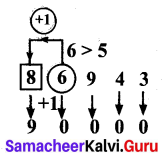(iv) To round off 5081739 to lakhs
The digit in the lakhs place is 0
The digit to the right is 8 > 5
Adding 1 to 0 ⇒ 1 + 0 = 1
Changing the digits right off 51 to zeros
We get 51,00,000
5081739 $$\simeq$$ 51,00,000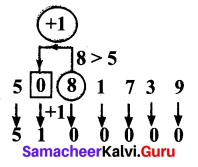(v) To round off 337598482 to ten crore
The digit in the ten crores place is 3
The digit to the right is 3 < 0
The digit in ten crore place remains the same 3.
Changing the digit to the right of 3 zeros we get 30,00,00,000
33,75,98,482 $$\simeq$$ 30,00,00,000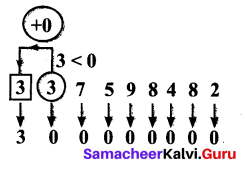Question 4.
Estimate the sum of 157826 and 32469 rounded off to the nearest ten thousand.
Solution: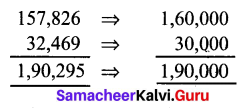Question 5.
Estimate by rounding off each number to the nearest hundred.
(i) 8074 + 4178
(ii) 1768977 + 130589
Solution: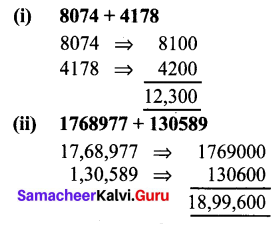Question 6.
The population of a city was 43,43,645 in the year 2001 and 46,81,087 in the year 2011. Estimate the increase in population by rounding off to the nearest thousand.
Solution:
Population in 2001 = 43,43,645
Population in 2011 = 46,81,087
Increase in population = 46,81,087 – 43,43,645 = 3,37,442
When rounded off to the nearest thousand = 3,37,000

Objective Type Questions

Question 7.
The number which on rounding off to nearest thousands given 11000 is
(a) 10345
(b) 10855
(c) 11799
(d) 10056
Solution:
(b) 10855
In 10855, the digit in a hundred places is 8 ≥ 5. So 11000Question 8.
The estimation to the nearest hundred of 76812 is
(a) 77000
(b) 76000
(c) 76800
(d) 76900
Solution:
(c) 76800

Question 9.
The number 9785764 is rounded off to nearest lakh as
(a) 9800000
(b) 9786000
(c) 9795600
(d) 9795000
Solution:
(a) 9800000
In ten thousand places, the digit is 8 5. So 9800000

Question 10.
The estimated difference of 167826 and 2765 rounded off to the nearest thousand is
(a) 180000
(b) 165000
(c) 140000
(d) 155000
Solution:
(b) 165000